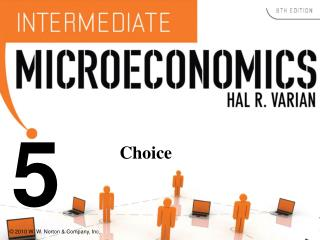Download PresentationChoice

Loading in 2 Seconds...

# Choice - PowerPoint PPT Presentation

5. Choice. Economic Rationality. The principal behavioral postulate is that a decisionmaker chooses its most preferred alternative from those available to it. The available choices constitute the choice set. How is the most preferred bundle in the choice set located?.I am the owner, or an agent authorized to act on behalf of the owner, of the copyrighted work described.
Download Presentation## Choice

An Image/Link below is provided (as is) to download presentation

Download Policy: Content on the Website is provided to you AS IS for your information and personal use and may not be sold / licensed / shared on other websites without getting consent from its author.While downloading, if for some reason you are not able to download a presentation, the publisher may have deleted the file from their server.

- - - - - - - - - - - - - - - - - - - - - - - - - - E N D - - - - - - - - - - - - - - - - - - - - - - - - - -
Presentation Transcript
1. 5 Choice

2. Economic Rationality • The principal behavioral postulate is that a decisionmaker chooses its most preferred alternative from those available to it. • The available choices constitute the choice set. • How is the most preferred bundle in the choice set located?

3. Rational Constrained Choice Utility x1

4. Rational Constrained Choice Utility x2 x1

5. Rational Constrained Choice Utility x2 x1

6. Rational Constrained Choice Utility x2 x1

7. Rational Constrained Choice Utility x2 x1

8. Rational Constrained Choice Utility x2 x1

9. Rational Constrained Choice Utility x2 x1

10. Rational Constrained Choice Utility Affordable, but not the most preferred affordable bundle. x2 x1

11. Rational Constrained Choice The most preferredof the affordable bundles. Utility Affordable, but not the most preferred affordable bundle. x2 x1

12. Rational Constrained Choice Utility x2 x1

13. Rational Constrained Choice Utility x2 x1

14. Rational Constrained Choice x2 Utility x1

15. Rational Constrained Choice x2 Utility x1

16. Rational Constrained Choice x2 Affordablebundles x1

17. Rational Constrained Choice x2 Affordablebundles x1

18. Rational Constrained Choice x2 More preferredbundles Affordablebundles x1

19. Rational Constrained Choice x2 More preferredbundles Affordablebundles x1

20. Rational Constrained Choice x2 x2* x1 x1*

21. Rational Constrained Choice x2 (x1*,x2*) is the mostpreferred affordablebundle. x2* x1 x1*

22. Rational Constrained Choice • The most preferred affordable bundle is called the consumer’s ORDINARY DEMAND at the given prices and budget. • Ordinary demands will be denoted byx1*(p1,p2,m) and x2*(p1,p2,m).

23. Rational Constrained Choice • When x1* > 0 and x2* > 0 the demanded bundle is INTERIOR. • If buying (x1*,x2*) costs \$m then the budget is exhausted.

24. Rational Constrained Choice x2 (x1*,x2*) is interior. (x1*,x2*) exhausts thebudget. x2* x1 x1*

25. Rational Constrained Choice x2 (x1*,x2*) is interior.(a) (x1*,x2*) exhausts thebudget; p1x1* + p2x2* = m. x2* x1 x1*

26. Rational Constrained Choice x2 (x1*,x2*) is interior .(b) The slope of the indiff.curve at (x1*,x2*) equals the slope of the budget constraint. x2* x1 x1*

27. Rational Constrained Choice • (x1*,x2*) satisfies two conditions: • (a) the budget is exhausted; p1x1* + p2x2* = m • (b) the slope of the budget constraint, -p1/p2, and the slope of the indifference curve containing (x1*,x2*) are equal at (x1*,x2*).

28. Computing Ordinary Demands • How can this information be used to locate (x1*,x2*) for given p1, p2 and m?

29. Computing Ordinary Demands - a Cobb-Douglas Example. • Suppose that the consumer has Cobb-Douglas preferences.

30. Computing Ordinary Demands - a Cobb-Douglas Example. • Suppose that the consumer has Cobb-Douglas preferences. • Then

31. Computing Ordinary Demands - a Cobb-Douglas Example. • So the MRS is

32. Computing Ordinary Demands - a Cobb-Douglas Example. • So the MRS is • At (x1*,x2*), MRS = -p1/p2 so

33. Computing Ordinary Demands - a Cobb-Douglas Example. • So the MRS is • At (x1*,x2*), MRS = -p1/p2 so (A)

34. Computing Ordinary Demands - a Cobb-Douglas Example. • (x1*,x2*) also exhausts the budget so (B)

35. Computing Ordinary Demands - a Cobb-Douglas Example. • So now we know that (A) (B)

36. Computing Ordinary Demands - a Cobb-Douglas Example. • So now we know that (A) Substitute (B)

37. Computing Ordinary Demands - a Cobb-Douglas Example. • So now we know that (A) Substitute (B) and get This simplifies to ….

38. Computing Ordinary Demands - a Cobb-Douglas Example.

39. Computing Ordinary Demands - a Cobb-Douglas Example. Substituting for x1* in then gives

40. Computing Ordinary Demands - a Cobb-Douglas Example. So we have discovered that the mostpreferred affordable bundle for a consumerwith Cobb-Douglas preferences is

41. Rational Constrained Choice • When x1* > 0 and x2* > 0 and (x1*,x2*) exhausts the budget,and indifference curves have no ‘kinks’, the ordinary demands are obtained by solving: • (a) p1x1* + p2x2* = y • (b) the slopes of the budget constraint, -p1/p2, and of the indifference curve containing (x1*,x2*) are equal at (x1*,x2*).

42. Rational Constrained Choice • But what if x1* = 0? • Or if x2* = 0? • If either x1* = 0 or x2* = 0 then the ordinary demand (x1*,x2*) is at a corner solution to the problem of maximizing utility subject to a budget constraint.

43. Examples of Corner Solutions -- the Perfect Substitutes Case x2 MRS = -1 Slope = -p1/p2 with p1 > p2. x1

44. Examples of Corner Solutions -- the Perfect Substitutes Case x2 MRS = -1 Slope = -p1/p2 with p1 > p2. x1

45. Examples of Corner Solutions -- the Perfect Substitutes Case x2 MRS = -1 Slope = -p1/p2 with p1 > p2. x1

46. Examples of Corner Solutions -- the Perfect Substitutes Case x2 MRS = -1 Slope = -p1/p2 with p1 < p2. x1